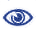# Prague Stringology Conference 2011

Analyzing Edit Distance on Trees: Tree Swap Distance is Intractable

 Abstract: The string correction problem looks at minimal ways to modify one string into another using fixed operations, such as for example inserting a symbol, deleting a symbol and interchanging the positions of two symbols (a “swap”). This has been generalized to trees in various ways, but unfortunately having operations to insert/delete nodes in the tree and operations that move subtrees, such as a “swap” of adjacent subtrees, makes the correction problem for trees intractable. In this paper we investigate what happens when we have a tree edit distance problem with only swaps. We call this problem tree swap distance, and go on to prove that this correction problem is NP-complete. This suggests that the swap operation is fundamentally problematic in the tree case, and other subtree movement models should be studied.

 Download paper:PostScript PDF BibTeX reference
 Download presentation: